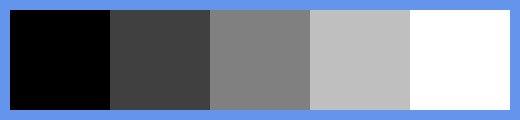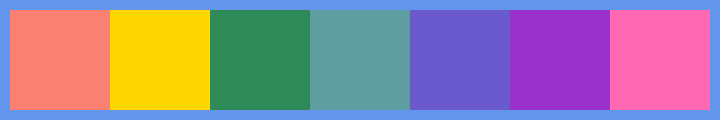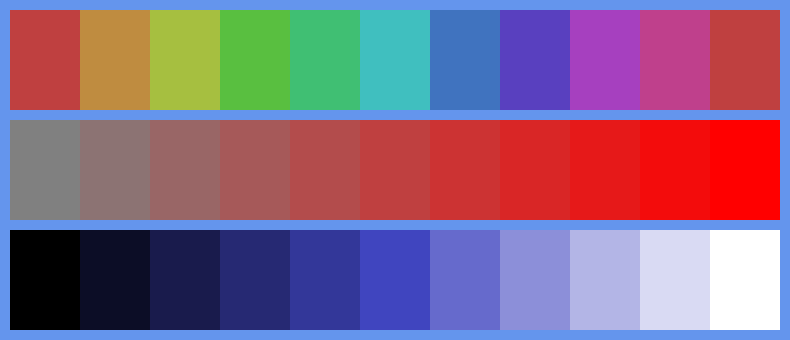# Colour spaces in generativepy

Martin McBride, 2021-11-10
Tags generativepy tutorial colour rgb hsl css colours
Categories generativepy generativepy tutorialgenerativepy provides several ways of defining colour values, via the color module. Colours are stored as Color objects. For more information about computer colour in general, see the colour articles in the computer science section.

You can create colours using:

• RGB values
• Grey values
• CSS named colours
• HSL values

## RGB colours

You can create a colour from RGB values using:

Color(r, g, b)


Where r, g and b are numbers. The supplied values will be clamped to the range 0.0 to 1.0.

For each colour of the values r, g, and b, a value of zero indicates that none of that colour is present, 0.5 means the colour is present at 50% of full intensity, 1.0 means that colour is present at full intensity.

Here is some code that creates a set of RGB colours and draws filled rectangles:

from generativepy.drawing import make_image, setup
from generativepy.color import Color
from generativepy.geometry import Rectangle

def draw_rgb(ctx, pixel_width, pixel_height, frame_no, frame_count):
setup(ctx, pixel_width, pixel_height, background=Color("cornflowerblue"))

pos = [10, 10]
w = 100
h = 100

Rectangle(ctx).of_corner_size(pos, w, h).fill(Color(0, 0, 0))
pos += w
Rectangle(ctx).of_corner_size(pos, w, h).fill(Color(0.5, 0, 0))
pos += w
Rectangle(ctx).of_corner_size(pos, w, h).fill(Color(1, 0, 0))
pos += w
Rectangle(ctx).of_corner_size(pos, w, h).fill(Color(0.5, 1, 0))
pos += w
Rectangle(ctx).of_corner_size(pos, w, h).fill(Color(0.5, 0, 1))
pos += w
Rectangle(ctx).of_corner_size(pos, w, h).fill(Color(0, 1, .5))
pos += w
Rectangle(ctx).of_corner_size(pos, w, h).fill(Color(0.5, 0.5, 0.5))
pos += w

make_image("colour-rgb.png", draw_rgb, 720, 120)


This code is available on github in tutorial/colour/colour_rgb.py.

All the images in this section have a background colour of cornflowerblue, set using a named colour as described later in the section. We can't use a white background because some of the colour patches, later on, will be white (we can't use a black or grey background for similar reasons). A distinct blue colour allows all the colour patches we use to show up.

Here is the image it creates:The images mix various amounts of red, green and blue to create different colours. You can use this code to experiment with other combinations.

## Grey colours

Mixing an equal amount of red, green and blue will give a grey colour. An rgb value of (0, 0, 0) gives black, (1, 1, 1) gives white, (0.2, 0.2, 0.2) gives a fairly dark grey, and so on.

As a convenience you can create a grey value like this:

Color(k)


This is effectively the same thing as Color(k, k, k).

This code creates a set of grey values from 0.0 (black) to 1.0 (white):

from generativepy.drawing import make_image, setup
from generativepy.color import Color
from generativepy.geometry import Rectangle

def draw_grey(ctx, pixel_width, pixel_height, frame_no, frame_count):
setup(ctx, pixel_width, pixel_height, background=Color('cornflowerblue'))

pos = [10, 10]
w = 100
h = 100

Rectangle(ctx).of_corner_size(pos, w, h).fill(Color(0))
pos += w
Rectangle(ctx).of_corner_size(pos, w, h).fill(Color(0.25))
pos += w
Rectangle(ctx).of_corner_size(pos, w, h).fill(Color(0.5))
pos += w
Rectangle(ctx).of_corner_size(pos, w, h).fill(Color(0.75))
pos += w
Rectangle(ctx).of_corner_size(pos, w, h).fill(Color(1))
pos += w

make_image("colour-grey.png", draw_grey, 520, 120)


This code is available on github in tutorial/colour/colour_grey.py.

Here is the image it creates:## CSS named colours

The CSS named colours are a set of over 150 colours that have been carefully chosen as generally pleasing colours. They were originally intended for use on websites, but they are also good for general design as well as certain types of generative art.

The set of colours includes some standard colours such as black, white, red, magenta etc. It also includes a large number of other colours across the spectrum.

You can create a colour from CSS name using:

Color("slateblue")


You must use a valid CSS name (you can find a list on various websites, or look at the color module source code). The string is case insensitive.

In addition, you can create variants of each colour by changing the brightness or saturation, as shown later.

This code creates a set of coloured squares using a selection of name colours:

from generativepy.drawing import make_image, setup
from generativepy.color import Color
from generativepy.geometry import Rectangle

def draw_css(ctx, pixel_width, pixel_height, frame_no, frame_count):
setup(ctx, pixel_width, pixel_height, background=Color("cornflowerblue"))

pos = [10, 10]
w = 100
h = 100

Rectangle(ctx).of_corner_size(pos, w, h).fill(Color("salmon"))
pos += w
Rectangle(ctx).of_corner_size(pos, w, h).fill(Color("gold"))
pos += w
Rectangle(ctx).of_corner_size(pos, w, h).fill(Color("seagreen"))
pos += w
pos += w
Rectangle(ctx).of_corner_size(pos, w, h).fill(Color("slateblue"))
pos += w
Rectangle(ctx).of_corner_size(pos, w, h).fill(Color("darkorchid"))
pos += w
Rectangle(ctx).of_corner_size(pos, w, h).fill(Color("hotpink"))
pos += w

make_image("colour-css.png", draw_css, 720, 120)


This code is available on github in tutorial/colour/colour_css.py.

Here is the image it creates:## HSL colours

HSL colours define colours based on hue, saturation and lightness:

• Hue defines the basic colour. A value from 0.0 to 1.0 represents the position of the colour on a colour circle.
• Saturation determines the "purity" of the colour. A saturation of 1.0 creates a pure colour. With lower saturation values, the colour appears more "greyed out" or pastel, but it is still identifiable as the same underlying colour. As the saturation approaches 0, the underlying colour vanishes and the result becomes grey.
• Lightness controls how light the colour is. Varying the lightness creates lighter or darker versions of the same colour. As the lightness approaches 0, the colour goes to black, and as the lightness approaches 1 the colour goes to white.

HSL is a remapping on RGB, which means that any colour you can create in RGB you can also create in HSL.

The advantage of HSL is that, once you have chosen a hue, you can easily create more or less saturated, and darker or lighter variants of the same colour. It is difficult to do that with RGB.

This code creates three lines of colour patches that vary in hue, saturation and brightness:

from generativepy.drawing import make_image, setup
from generativepy.color import Color
from generativepy.geometry import Rectangle

def draw_hsl(ctx, pixel_width, pixel_height, frame_no, frame_count):
setup(ctx, pixel_width, pixel_height, background=Color('cornflowerblue'))

pos = [10, 10]
w = 70
h = 100

Rectangle(ctx).of_corner_size(pos, w, h).fill(Color.of_hsl(0.0, 0.5, 0.5))
pos += w
Rectangle(ctx).of_corner_size(pos, w, h).fill(Color.of_hsl(0.1, 0.5, 0.5))
pos += w
Rectangle(ctx).of_corner_size(pos, w, h).fill(Color.of_hsl(0.2, 0.5, 0.5))
pos += w
Rectangle(ctx).of_corner_size(pos, w, h).fill(Color.of_hsl(0.3, 0.5, 0.5))
pos += w
Rectangle(ctx).of_corner_size(pos, w, h).fill(Color.of_hsl(0.4, 0.5, 0.5))
pos += w
Rectangle(ctx).of_corner_size(pos, w, h).fill(Color.of_hsl(0.5, 0.5, 0.5))
pos += w
Rectangle(ctx).of_corner_size(pos, w, h).fill(Color.of_hsl(0.6, 0.5, 0.5))
pos += w
Rectangle(ctx).of_corner_size(pos, w, h).fill(Color.of_hsl(0.7, 0.5, 0.5))
pos += w
Rectangle(ctx).of_corner_size(pos, w, h).fill(Color.of_hsl(0.8, 0.5, 0.5))
pos += w
Rectangle(ctx).of_corner_size(pos, w, h).fill(Color.of_hsl(0.9, 0.5, 0.5))
pos += w

pos = [10, 120]
Rectangle(ctx).of_corner_size(pos, w, h).fill(Color.of_hsl(0.0, 0.0, 0.5))
pos += w
Rectangle(ctx).of_corner_size(pos, w, h).fill(Color.of_hsl(0.0, 0.1, 0.5))
pos += w
Rectangle(ctx).of_corner_size(pos, w, h).fill(Color.of_hsl(0.0, 0.2, 0.5))
pos += w
Rectangle(ctx).of_corner_size(pos, w, h).fill(Color.of_hsl(0.0, 0.3, 0.5))
pos += w
Rectangle(ctx).of_corner_size(pos, w, h).fill(Color.of_hsl(0.0, 0.4, 0.5))
pos += w
Rectangle(ctx).of_corner_size(pos, w, h).fill(Color.of_hsl(0.0, 0.5, 0.5))
pos += w
Rectangle(ctx).of_corner_size(pos, w, h).fill(Color.of_hsl(0.0, 0.6, 0.5))
pos += w
Rectangle(ctx).of_corner_size(pos, w, h).fill(Color.of_hsl(0.0, 0.7, 0.5))
pos += w
Rectangle(ctx).of_corner_size(pos, w, h).fill(Color.of_hsl(0.0, 0.8, 0.5))
pos += w
Rectangle(ctx).of_corner_size(pos, w, h).fill(Color.of_hsl(0.0, 0.9, 0.5))
pos += w

pos = [10, 230]
Rectangle(ctx).of_corner_size(pos, w, h).fill(Color.of_hsl(0.66, 0.5, 0.0))
pos += w
Rectangle(ctx).of_corner_size(pos, w, h).fill(Color.of_hsl(0.66, 0.5, 0.1))
pos += w
Rectangle(ctx).of_corner_size(pos, w, h).fill(Color.of_hsl(0.66, 0.5, 0.2))
pos += w
Rectangle(ctx).of_corner_size(pos, w, h).fill(Color.of_hsl(0.66, 0.5, 0.3))
pos += w
Rectangle(ctx).of_corner_size(pos, w, h).fill(Color.of_hsl(0.66, 0.5, 0.4))
pos += w
Rectangle(ctx).of_corner_size(pos, w, h).fill(Color.of_hsl(0.66, 0.5, 0.5))
pos += w
Rectangle(ctx).of_corner_size(pos, w, h).fill(Color.of_hsl(0.66, 0.5, 0.6))
pos += w
Rectangle(ctx).of_corner_size(pos, w, h).fill(Color.of_hsl(0.66, 0.5, 0.7))
pos += w
Rectangle(ctx).of_corner_size(pos, w, h).fill(Color.of_hsl(0.66, 0.5, 0.8))
pos += w
Rectangle(ctx).of_corner_size(pos, w, h).fill(Color.of_hsl(0.66, 0.5, 0.9))
pos += w

make_image("colour-hsl.png", draw_hsl, 720, 340)


This code is available on github in tutorial/colour/colour_hsl.py.

Here is the image it creates:To give a little more explanation, the first row has varying hues:

• Hue 0 is red.
• As the hue value increases the colour gradually changes to yellow then to green.
• Hue 0.333 is green.
• As the hue value increases the colour gradually changes to cyan then to blue.
• Hue 0.666 is blue.
• As the hue value increases the colour gradually changes to magenta then back to red.
• Hue is a cyclical quantity. As it moves from 0 to 1 it completes a full red-green-blue colour circle and ends up back at red.

The second row has varying saturation. Each patch has the same red hue, but it changes from grey to more saturated red as the saturation increases from 0 to 1.

The third row has varying lightness. Each patch has the same blue hue, but it changes from black to a lighter and lighter blue, eventually moving towards white as lightness increases.

If you found this article useful, you might be interested in the book Computer Graphics in Python or other books by the same author.

#### Popular tags

2d arrays abstract data type alignment and angle animation arange arc array arrays behavioural pattern bezier curve built-in function callable object chain circle classes clipping close closure cmyk colour combinations comparison operator comprehension context context manager conversion count creational pattern data science data types design pattern device space dictionary drawing duck typing efficiency ellipse else encryption enumerate fill filter font font style for loop function function composition function plot functools game development generativepy tutorial generator geometry gif global variable gradient greyscale higher order function hsl html image image processing imagesurface immutable object in operator index inner function input installing iter iterable iterator itertools join l system lambda function len line linear gradient linspace list list comprehension logical operator lru_cache magic method mandelbrot mandelbrot set map matplotlib monad mutability named parameter numeric python numpy object open operator optimisation optional parameter or pandas partial application path pattern permutations polygon positional parameter print pure function python standard library radial gradient range recipes rectangle recursion reduce repeat rgb rotation roundrect scaling scipy sector segment sequence setup shape singleton slice slicing sound spirograph sprite square str stream string stroke structural pattern subpath symmetric encryption template text text metrics tinkerbell fractal transform translation transparency triangle truthy value tuple turtle unpacking user space vectorisation webserver website while loop zip